# A horizontal wire of length 1.0 m carries a current of 48 A to the right....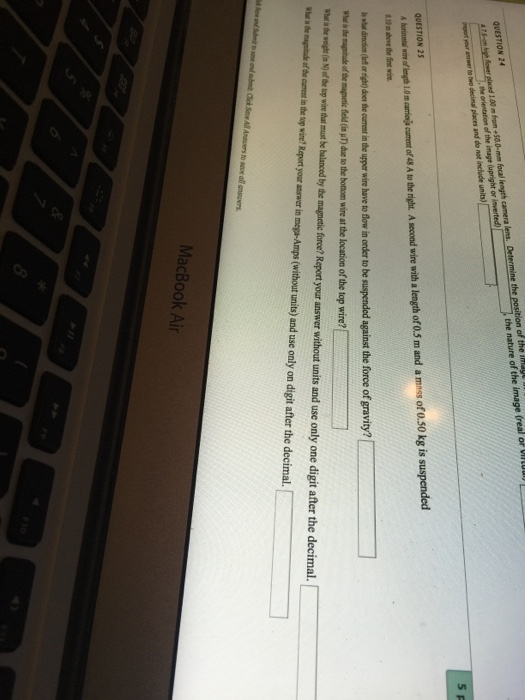A horizontal wire of length 1.0 m carries a current of 48 A to the right. A second wire with a length of 0.5 m and a mass of 0.50 kg is suspend 0.30 m above the first wire. In what direction(left or right) does the current in the upper wire have to flow in order to be suspended against the force of gravity? What is the magnitude of the magnetic field (in mu T) due to the bottom wire at the location of the top wire? What the weight (in N) of the lop wire that must be balanced by the magnetic force? Report your answer without units and use only digit after the decimal. What is the magnitude of the current in the top wire? Report your answer in mega-Amps (without units) and use only on digit after the decimal.

length of horizontal wire = 1m ; current ( left to right ) =48A

second wire length =0.5m ; mass=0.5Kg ; height from 1st wire =0.30 m

(a) direction of current in second suspended wire = From Right to left ( because conductors carrying current in opposite directions repel each other)

(b) field at upper wire due to bottom wire

i = current in first wire r = distance of second wire from first

= permeability constant = 1.26 X 10^(-6) henry/m

B = 1.26 X 10^(-6) H/m X 48 A / 2 X 3.14 X 0.3 m = 32.1

(c) weight of the top wire that must be balanced = m g = 0.5 Kg X 9.8m/s^2 = 4.9 N

(d) Current in top wire to balance weight = ?

F = 4.9 N ( to balance wight of top wire) is required to be created at top wire due to bottom wire. This should be repulsive force

where l = length of 2nd wire at top

= current in wire at bottom , = current in top wire d= distance between the wires

F = 1.26 X 10^(-6) H/m X 0.5 m X 48 A X /(2) = 4.9N

= 2 X 3.14 X 0.3 m X 49 N /( 1.26 X 10^(-6) X ).5 X 48 A) = 3.0 X 10^(6) A

##### Add Answer to: A horizontal wire of length 1.0 m carries a current of 48 A to the right....
Similar Homework Help Questions
• ### The wire in the figure carries a current of 2.22 A and has a length of...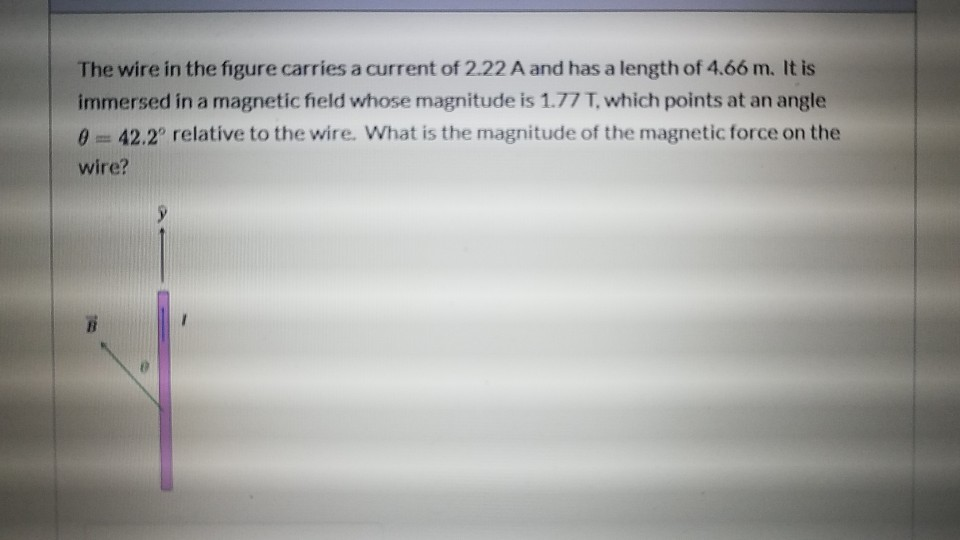The wire in the figure carries a current of 2.22 A and has a length of 4.66 m. It is immersed in a magnetic field whose magnitude is 1.77 T which points at an angle of 42.2° relative to the wire. What is the magnitude of the magnetic force on the wire? The wire in the figure carries a current of 2.22 A and has a length of 4.66 m. It is immersed in a magnetic field whose magnitude is...

• ### A wire of 1.0 m length carries the current I1 = 25 A. Another wire of...

A wire of 1.0 m length carries the current I1 = 25 A. Another wire of the same length, parallel to the first one carries the current I2 = 15 A. As a result, the wires attract each other with the force F = 1.5 mN. What is the distance between the wires? Are the currents of the same or opposite directions? What of the following statements is/are correct? A. The current in one wire create electric field, which excerts...

• ### A wire of 1.0 m length carries the current I1 = 25 A. Another wire of...

A wire of 1.0 m length carries the current I1 = 25 A. Another wire of the same length, parallel to the first one carries the current I2 = 15 A. As a result, the wires attract each other with the force F = 1.5 mN. What is the distance between the wires? Are the currents of the same or opposite directions? What of the following statements is/are correct? A. The current in one wire create electric field, which exerts...

• ### 4. A horizontal wire of length 3.0 m carries a current of 6.0 A and is...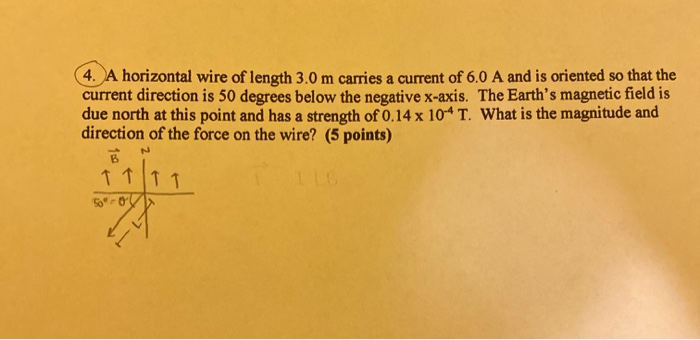4. A horizontal wire of length 3.0 m carries a current of 6.0 A and is oriented so that the current direction is 50 degrees below the negative x-axis. The Earth's magnetic field is due north at this point and has a strength of 0.14 x 10-4 T. What is the magnitude and direction of the force on the wire? (5 points) B LB N ↑ ↑ TT 50-0

• ### 3. A straight wire of mass 200 g and length 1.5 m carries a current of...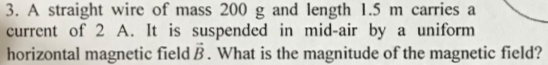3. A straight wire of mass 200 g and length 1.5 m carries a current of 2 A. It is suspended in mid-air by a uniform horizontal magnetic field B. What is the magnitude of the magnetic field?

• ### The wire in the figure carries a current of 2.22 A and has a length of...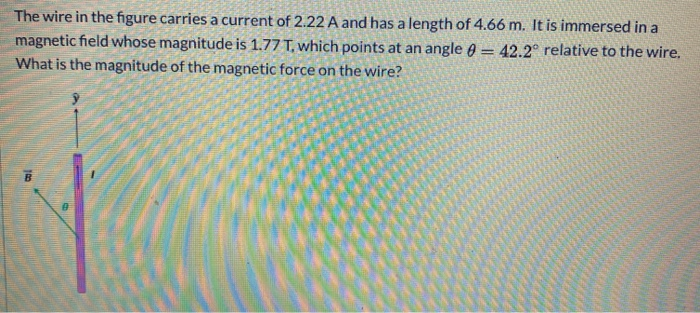The wire in the figure carries a current of 2.22 A and has a length of 4.66 m. It is immersed in a magnetic field whose magnitude is 1.77 T, which points at an angle A = 42.2 relative to the wire. What is the magnitude of the magnetic force on the wire? TO

• ### The wire in the figure carries a current of 2.22 A and has a length of...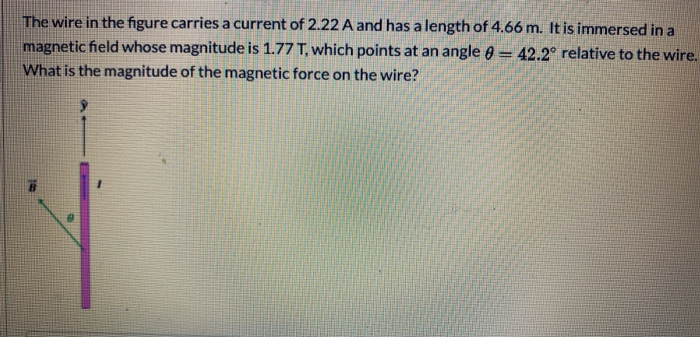The wire in the figure carries a current of 2.22 A and has a length of 4.66 m. It is immersed in a magnetic field whose magnitude is 1.77 T, which points at an angle 8 = 42.2º relative to the wire. What is the magnitude of the magnetic force on the wire?

• ### The wire in the figure carries a current of 2.22 A and has a length of...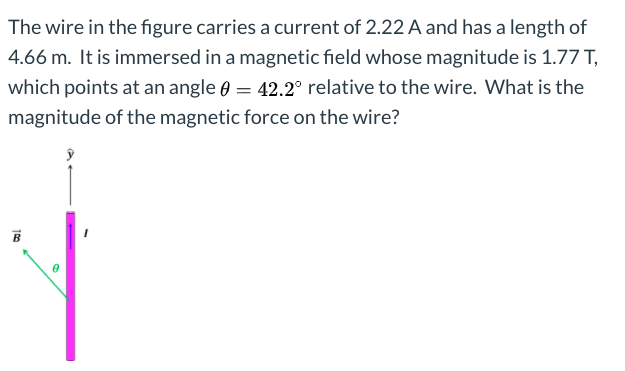The wire in the figure carries a current of 2.22 A and has a length of 4.66 m. It is immersed in a magnetic field whose magnitude is 1.77 T, which points at an angle 6 = 42.2º relative to the wire. What is the magnitude of the magnetic force on the wire? 100

• ### 2. (15 points) A wire of 1.0 m length carries the current I, = 25 A....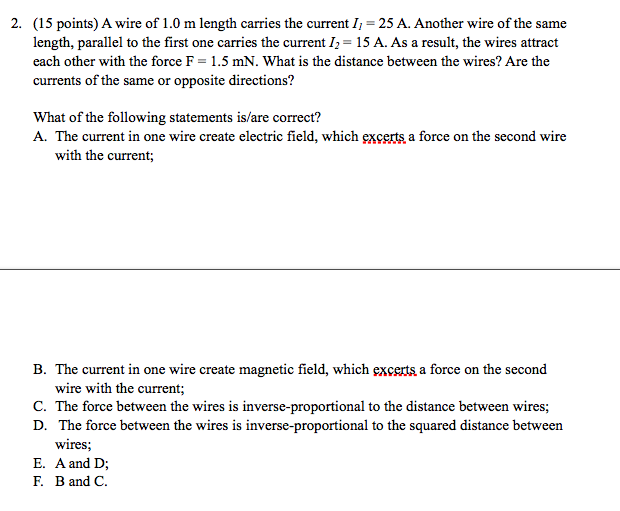2. (15 points) A wire of 1.0 m length carries the current I, = 25 A. Another wire of the same length, parallel to the first one carries the current Iz = 15 A. As a result, the wires attract each other with the force F = 1.5 mN. What is the distance between the wires? Are the currents of the same or opposite directions? What of the following statements is/are correct? A. The current in one wire create electric...

• ### 2. (15 points) A wire of 1.0 m length carries the current I1 = 25 A....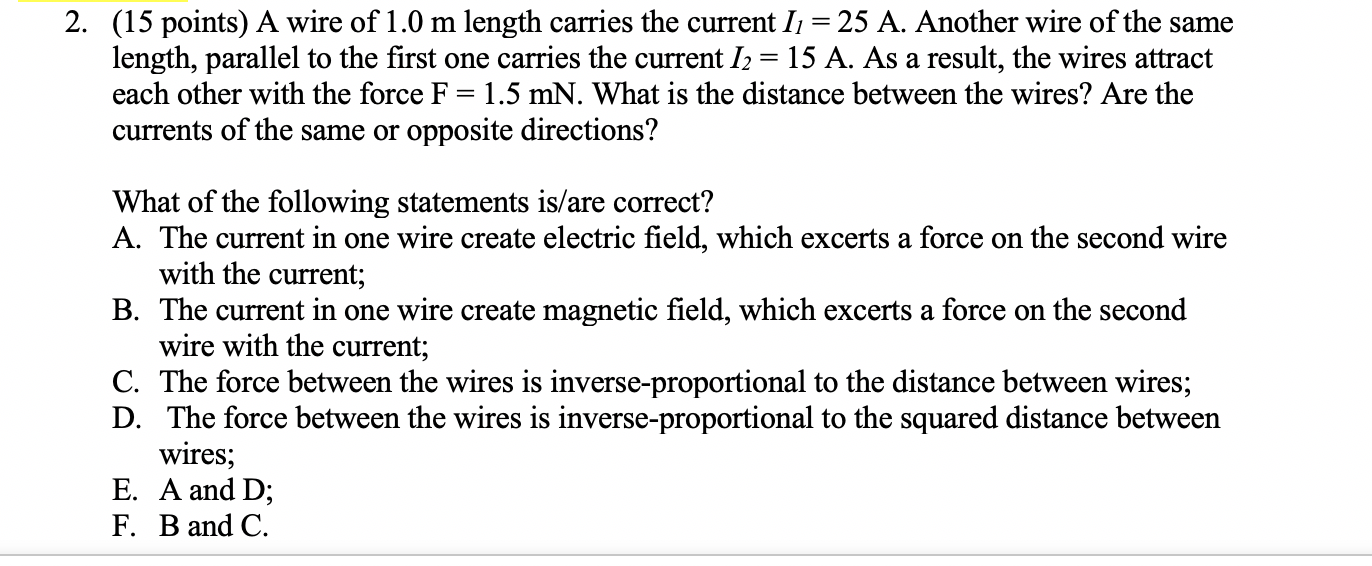2. (15 points) A wire of 1.0 m length carries the current I1 = 25 A. Another wire of the same length, parallel to the first one carries the current 12 = 15 A. As a result, the wires attract each other with the force F= 1.5 mN. What is the distance between the wires? Are the currents of the same or opposite directions? What of the following statements is/are correct? A. The current in one wire create electric field,...

Free Homework App